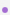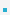11. Void Pointers and Function Pointers

11.1 Void PointersC does not allow us to declare and use void variables.void can be used only as return type or parameter of a function.C allows void pointersQuestion: What are some scenarios where you want to pass void pointers?void pointers can be used to point to any data type

 int x; void ∗p = &x;          /∗ points to int ∗/ float f; void ∗p = &f;        /∗ points to float ∗/void pointers cannot be dereferenced. The pointers should always be cast before dereferencing.

 void ∗p; printf ("%d", ∗p); /∗ invalid ∗/ void ∗p; int ∗px = (int ∗)p; printf ("%d", ∗px); /∗ valid ∗/

11.2 Function PointersIn C, function itself is not a variable. But it is possible to declare pointer to functions.Function pointers can be assigned, pass to and from functions, placed in arrays etc.Question: What are some scenarios where you want to pass pointers to functions?Declaration examples:

 int (∗fp )(int)                 /∗ notice the () ∗/ int (∗fp )(void∗, void∗)

Example: Function Pointer
 /* The four arithmetic operations ... one of these functions is selected at runtime with a swicth or a function pointer */ float plus (float a, float b) { return a+b; } float minus (float a, float b) { return a-b; } float multiply(float a, float b) { return a*b; } float divide (float a, float b) { return a/b; } void switch(float a, float b, char opCode) {     float result;     switch(opCode)     {         case '+' : result = plus(a, b);           break;         case '-' : result = minus(a, b); break;         case '*' : result = multiply(a, b);      break;         case '/' : result = divide (a, b);        break;     }     printf(“%f\n”, result); } /* Solution with a function pointer */ void switch_pointer           (float a, float b, float(*pt2func)(float, float)) {     float result = pt2func(a, b); /* call using function pt */     printf(“result=%f\n”, result); } /* Execute example code */ void switch2() {     switch(2, 5, ‘+’);     switch_pointer(2, 5, &minus);}

11.3 CallbackDefinition: Callback is a piece of executable code passed to functions. In C, callbacks are implemented    by passing function pointers.

 #include #include /* The calling function takes a single callback as a parameter. */ void PrintTwoNumbers(int (*numberSource)(void)) {     printf("%d and %d\n", numberSource(), numberSource()); } /* One possible callback. */ int overNineThousand(void) {     return (rand() % 1000) + 9000; } /* Another possible callback. */ int fortyTwo(void) {     return 42; } /* Here we call PrintTwoNumbers() with three different callbacks. */ int main(void) {     PrintTwoNumbers(rand);     PrintTwoNumbers(overNineThousand);     PrintTwoNumbers(fortyTwo); }

 이전페이지 /12/ 다음페이지
000